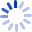# Modern Control Engineering

FEATURES: *Emphasizes the basic concepts and the design aspects of control systems. *Avoids highly mathematical arguments but provides mathematical proofs whenever they contribute to an understanding of the material. *Includes examples at strategic points throughout (over 100 total). *Contains numerous worked problems (A-problems) at the end of each chapter (over 200 total), and 200 unsolved problem (B-problems). *Provides introductory discussion on the two-degrees-of-freedom control system, and introduces design conditions ...

##### Cash for Textbooks